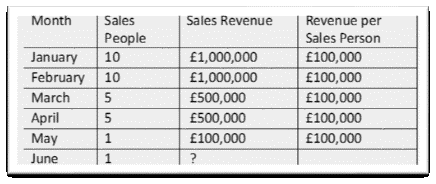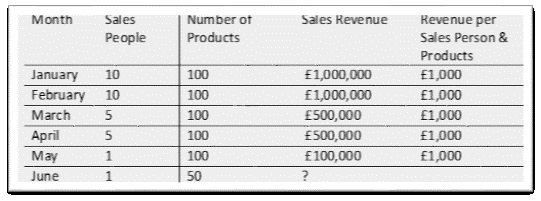# Regression Forecasting Explained in 500 Words

Regression, or rather regression analysis, is a standard and common statistical method of forecasting. For some reason, probably because of the name ‘regression’ itself, people often perceive it to be more complicated than it actually is.

Whilst undertaking regression analysis can often be complex, the concept itself is actually very straightforward. If you have ever wondered what it is, or how to do it, here is my explanation of the concept of regression.

In essence, regression comes in two forms; either simple regression or multiple regression.

## Simple Regression

Simple regression is based on two variables that are related, with one variable forecasting another. To put that into real world terms, let’s say that your sales revenue has a direct relationship with the number of sales people you have in the field.

If you look at your sales revenue history, and the number of sales people working, you may see a relationship as in the table below:If you divide the revenue by the number of sales people each month, then it is clear to see that for each sales person £100,000 revenue is generated each month. Consequently, it is very easy to forecast June’s sales revenue based on 1 sales person. The answer is of course £100,000, and that in a nut-shell, is simple regression.

## Multiple Regression

Multiple regression is where you use multiple variables to forecast another. So again, using the same example, but this time also including the number of products available for sale we get the following:In this example the relationship between sales people, number of products and revenue stays the same each month. However, in June you can see that the number of products available drops to 50. Consequently the forecast revenue for June will not be £100,000 as we predicted with simple regression, but rather £50,000 based on the historical relationship of sales people AND number of products. So we have forecasted using two variables to predict another, and that is multiple regression.

Of course, these are simplified examples aimed at explaining the concept of regression in forecasting. Undertaking regression analysis is often a much more involved and complex statistical affair, but for now I hope this brief article has given you an understanding of the regression concept.